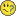# New to QlikView

Discussion board where members can get started with QlikView.

Announcements
Save the Date: QlikWorld Online, June 24-25, 2020. Free global virtual event for data integration and data analytic gurus. Register Today
Contributor

## ValueList - Expression Problem

I'm sure there is a simple solution to my problem, I just can't work out what it is

I have written an expression using a ValueList, which is working fine for the first two parameters on the list.  When I try to add the third value it fails (the column shows nulls throughout).  Can anyone see what I am doing wrong?   I have removed the calculation, and just put in a simple '1' result, just to try and get the value to show on the table - at the moment I can only see the first two values (Ambient and Multi Temp)Can anyone help?

Thank you

1 Solution

Accepted Solutions
HighlightedMVP

## Re: ValueList - Expression Problem

Hi Leah, after the ActWsvD there is and extra ')', remove it, you also need to remove the last ',)' line and set '))' at the end

10 Replies
HighlightedMVP

## Re: ValueList - Expression Problem

Hi Leah, after the ActWsvD there is and extra ')', remove it, you also need to remove the last ',)' line and set '))' at the end

Highlighted
Contributor

## Re: ValueList - Expression Problem

That worked a treat

Thank you RubenHighlighted
Valued Contributor II

## Re: ValueList - Expression Problem

Further to Ruben's answer, you may want to experiment with using the Pick function as I feel it makes it easier to read and maintain the varying expressions. There are two methods ...

The first one is to order your expressions in reverse alphabetical rank order ...

=pick(rank(valuelist('Ambient','Multi Temp','Central Billing','Total')),
'Total Expr',
//Expression to match last alphabetical rank
'Multi Temp Expr', // .. order expressions in reverse order
'Central Billing Expr', // .. as per alphabetical rank result
'Ambient Expr' //Expression to match first alphabetical rank
)

The second is to assign a numerical value to the Valuelists and change your dimension to match, resulting in the expressions listed in the correct order ...

Dimension:

=valuelist(Dual('Ambient',1),Dual('Multi Temp',2),Dual('Central Billing',3),Dual('Total',4))

Expression:

=pick(valuelist(Dual('Ambient',1),Dual('Multi Temp',2),Dual('Central Billing',3),Dual('Total',4)),
'Ambient Expr',
'Multi Temp Expr',
'Central Billing Expr',
'Total Expr'
)

Hope these help

flipside

Highlighted
Contributor

## Re: ValueList - Expression Problem

Thanks Flipside.

I've not used the PICK function before, and I'm always looking for ways to make processes simpler to maintain (aren't we all?!).

I appreciate your inputHighlighted
Valued Contributor II

## Re: ValueList - Expression Problem

Using this method will help negate the comma and bracket confusion associated with nested ifs. It's great when you revisit very complex expressions that need tweaking months or years after being first written.

Highlighted
Contributor

## Re: ValueList - Expression Problem

Hi,

I am having a unique issue while using valuelist() in a straight table that I am unable to find any help with:

My Dimension:

Valuelist('A','B','C')

My Expression:

pick(match(valuelist('A','B','C')

'A','B','C')

,sum(aggr(if((min({<planyear_endyear={\$(=max(planyear_endyear)-1)}>} salesfigure)) > 10000, 1,0), storeID))

,sum(aggr(if((min({<planyear_endyear={\$(=max(planyear_endyear)-1)}>} salesfigure)) < 10000, 1,0), storeID))

,sum(aggr(if((min({<planyear_endyear={\$(=max(planyear_endyear)-1)}>} salesfigure)) = 0, 1,0), storeID))

)

But the Result is coming out to be:

A     54

B     0

C     0

No matter in which order I keep my 3 formulas, only the first one works and rest of them comes out as zero. However, if I create three text boxes and put these 3 formulas in each of these text boxes, I can see the results of all three formulas. (I am scratching my head) Please help.

Thank you,

Highlighted
Contributor

## Re: ValueList - Expression Problem

Try it without the match:

pick(valuelist('A','B','C'),

sum(aggr(if((min({<planyear_endyear={\$(=max(planyear_endyear)-1)}>} salesfigure)) > 10000, 1,0), storeID)),

sum(aggr(if((min({<planyear_endyear={\$(=max(planyear_endyear)-1)}>} salesfigure)) < 10000, 1,0), storeID)),

sum(aggr(if((min({<planyear_endyear={\$(=max(planyear_endyear)-1)}>} salesfigure)) = 0, 1,0), storeID))

)

Highlighted
Contributor

## Re: ValueList - Expression Problem

Thanks Leah, I tried but it didn't work. The results are coming out as

A     -

B     -

C     -

Highlighted
Contributor

## Re: ValueList - Expression Problem

Okay ....try this:

pick(valuelist('A','B','C'),

(sum(aggr(if((min({<planyear_endyear={\$(=max(planyear_endyear)-1)}>} salesfigure)) > 10000, 1,0), storeID))),

(sum(aggr(if((min({<planyear_endyear={\$(=max(planyear_endyear)-1)}>} salesfigure)) < 10000, 1,0), storeID))),

(sum(aggr(if((min({<planyear_endyear={\$(=max(planyear_endyear)-1)}>} salesfigure)) = 0, 1,0), storeID)))

)